In this tutorial, we will learn about how to plot Bar Charts using the `Pandas` library.

Pandas library provides us with a way to plot different kinds of charts out of the data frames that are easy to understand and get insights about the data. Pandas library uses the `matplotlib` as default backend which is the most popular plotting module in python.

## 1. What is a Bar Chart

As the name suggests a bar chart is a chart showing the discrete values for different items as bars whose length is proportional to the value of the item and a bar chart can be vertical or horizontal. Usually, the x-axis is taken as the six to show different items of the data and the y-axis shows their values on the same scale such that their values are easily comparable.

## 2. Plotting Bar Charts in Pandas

The first step is to import the required libraries and load the data that we will be working upon. For this tutorial, we will be using the dataset that contains the India population data from the census that held once in every 10 years. The dataset can be found here. See the code below to execute these steps. All the steps are performed in Jupyter Notebooks.

Let us import the necessary libraries and load the data and see how it looks like

``````#import Libraries
import pandas as pd

Output: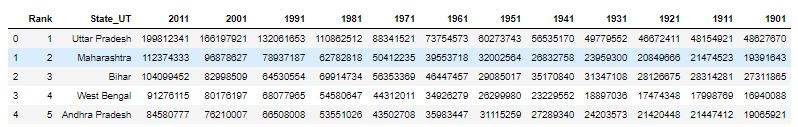## 2.1 Plotting vertical bar chart with multicoloured bars

Let us plot a bar chart that will show the population of India as recorded in the year 2011 and plot the top 5 most populated cities in descending order and all having different colours for bars. See the code below to implement this.

``````top5 = data.iloc[:5,:]
chart1 = top5.plot(kind='bar',x="State_UT",figsize=(6,6),y=2011,color=['cyan', 'red', 'orange', 'yellow', 'blue'])``````

Output: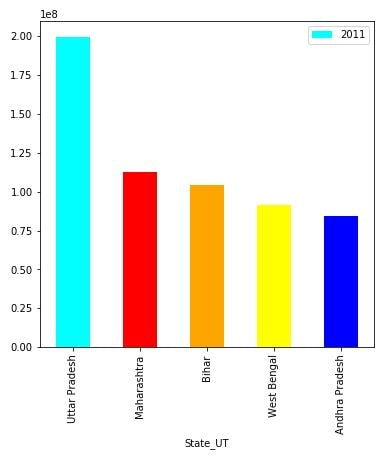## 2.1 Plotting horizontal bar chart comparing multiple columns

We can also plot a bar chart where bars are positioned horizontally on the y-axis, this can be achieved by using the `kind` argument and set it to “barh”. Also for each value of the item or state(in our example), we can plot bars for multiple columns in a single bar chart. See the following code to implement this.

``````chart2 = top5.plot(kind='barh',x="State_UT",figsize=(6,6),y=[2011,2001,1991]
,color=['brown', 'green', 'yellow'])``````

Output: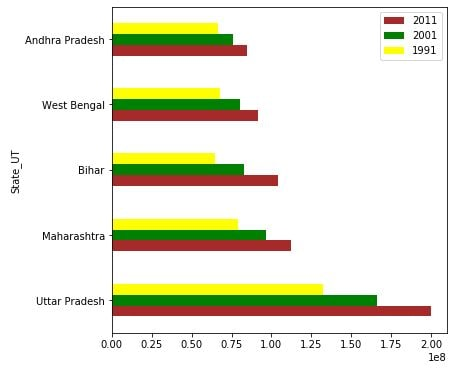### Plotting Stacked bar charts

The Stacked bar charts are made by stacking the value or bars for each column on top of each other. It is greatly used in time series data for comparing the percentage change over years, etc. We have to use the` stacked` argument and set it to True to achieve stacking. Let us plot a simple stacked bar chart for 3 columns from our data. That shows the population difference in 10 years. See the below code for implementation.

``````# Data preprocessing
pop_change = top5.iloc[:,:6]
pop_change["2001 - 2011"] = pop_change-pop_change
pop_change["1991 - 2001"] = pop_change-pop_change
pop_change["1981 - 1991"] = pop_change-pop_change
pop_change.drop([1981,1991,2011,2001],axis=1)``````

Output: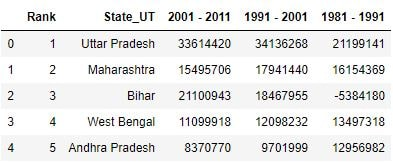``````# Plotting
chart4 = pop_change.plot.bar(x="State_UT",figsize=(6,6),
y=["2001 - 2011","1991 - 2001","1981 - 1991"],
stacked=True, fontsize=15,rot=45)``````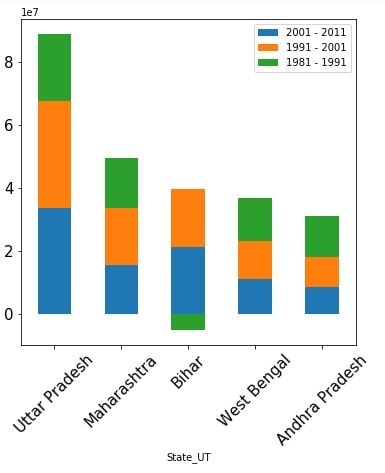So, We have learned how to plot bar charts out of pandas data-frames and matplotlib as backend. If you have any doubt, feel free to ask in the comment section below.

Happy Learning 🙂